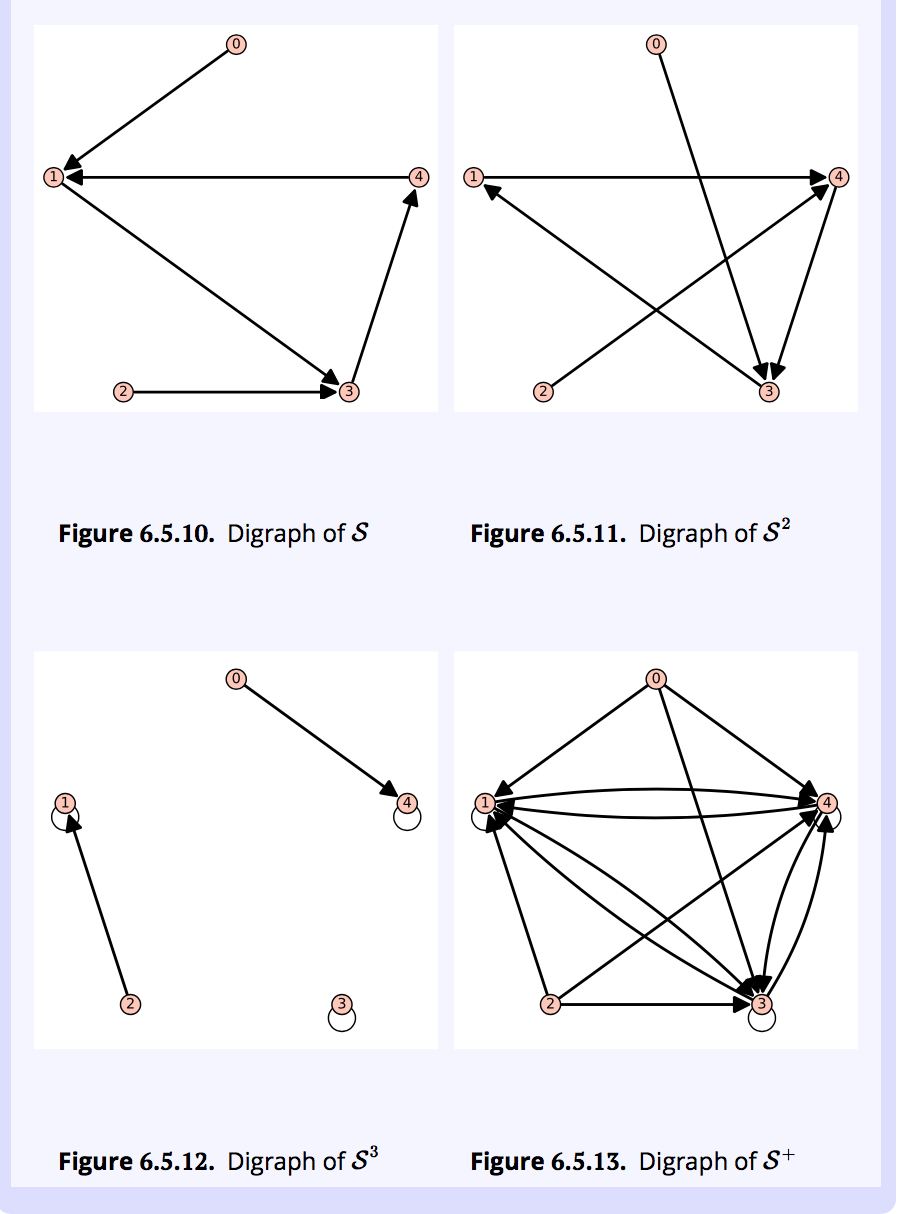# Errata - Applied Discrete Structures - V3.3

As significant mathematics errors appear, they will be logged here. Most of the typographical errors (missing/extraneous spaces, mathematics in non-math font, etc.) are not listed.

## Version 3.3 - May 2017

• Section 1.5, Exercise 8 should read "For any positive integer $k$, let $A_k = \dots$" (found by Matthew Haner)
• In the proof of Theorem 7.2.11 - The Pigeonhole Principle: The fourth sentence should end with "...form a partition of X."
• Section 6.5: In the pdf and print versions, the figures for the solution to exercise 3 of Section 6.5 are missing. They are• The definition of subgraph in Section 9.1 needs clarification. Here is an interim definition
Definition: Subgraph

Let $G=(V,E)$ be a graph of any kind: directed, directed multigraph, or undirected. $G'=(V',E')$ is a subgraph of $G$ if $V' \subseteq V$ and $e \in E'$ only if $e \in E$ and the vertices of $e$ are in $V'$. You create a subgraph of $G$ by removing zero or more vertices and all edges that include the removed vertices and then you possibly remove some other edges.

If the only removed edges are those that include the removed vertices, then we say that $G$ is an induced subgraph. Finally, $G'$ is a spanning subgraph of $G$ if $V' = V$, or, in other words, no vertices are removed from $G$, only edges.

• Section 9.1, exercise 9: Solution to part b should end with "... is a cycle of length 6." (found by Shelbylynn McCoy)
• 9.3.4 Sage Note: In the paragraph between the third and fourth SageMath cells, the second sentence should read "The expression Gr.depth_first_search(0) creates an iterator ... ." (found by Alexa Hyde)
• Section 11.4: The first line of the body of Table 11.4.10 should be
 -- 152 1 0
• Section 16.5: The statement of Theorem 16.5.6 is incorrect. It should be
Let $[F; +, \cdot ]$ be a field. Polynomial $f(x)$ is a unit in $F[x]$ if and only if it is a nonzero constant polynomial.

Errata for V2.0----Errata for V3.0----Errata for V3.2